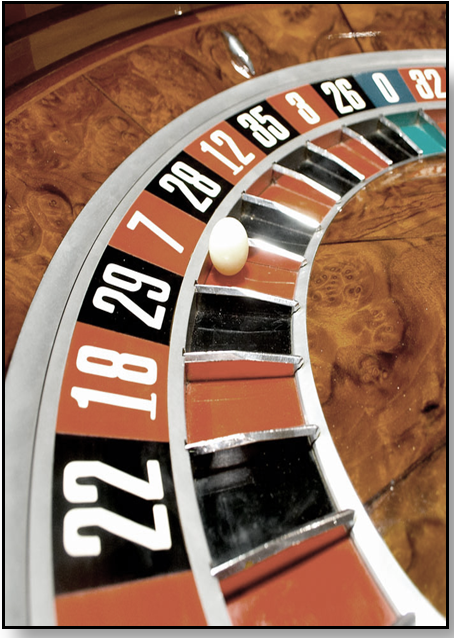## Tuesday, April 22, 2014

### Random IdeasAmong the many obscure functions in Excel is the useful and versatile RAND function. Each time your worksheet recalculates, (by reopening or forced recalculation by pressing F9), the RAND function returns a new Random Number that is greater than or equal to 0 and less than 1.  The syntax for the Rand function is simply:  =RAND()

So, what are some thoughts on using this interesting function?  Well, how you use it is only limited by your imagination, but here are a few examples of Random Ideas:

·       Use =RAND()*100 to return random numbers between 1 and 100

·       Or =RAND()*100 + 100 to return random numbers between 100 and 200

·       If you want only whole numbers, use:
=INT(RAND()*100+100 to return integers between 100 and 200

Or consider this, let’s say you have a database of 1,000 records from which you want to take a random sample of 15% of the data.  If your data is, for instance, in Column ‘A’, put in the following formula in Column ‘B’, and Autofill it to the end of data field:

=RAND()<=0.15

Then simply sort your columns based on Column B and retain the first 150 of your data field.  This will, of course, change every time the worksheet is recalculated because RAND is, well, Random!

Some extremely clever Excel Enthusiasts have created some amazing games using RAND.  For my own part, (modest in comparison to what some others have done), I have used the RAND function (along with other functions and graphics), to create a Vegas-style Slot Machine in Excel.  If you are interested, please send me a request at ExcelEnthusiasts@gmail.com if you would like a copy of the Slot Machine workbook, and I will be happy to send it to you.

By the way, if you have any RANDom Ideas regarding this cool function, I would love to hear about them!

#### 1 comment:

BobJordanB said...

Just to add to this one. The rand() function produces a uniform random number. What if you wantyed a rendom Normal number?

It is quite easy - use =Norminv(rand(),Mn,SD)

where Mn is the mean of the intended distribution and SD its standard deviation.

There are other distributions in Excel you can use to form other random variates too

Bob JordanB